• Math.NET过滤 Math.NET过滤是一种数字信号处理工具包，提供了用于数字滤波器设计的基础结构，可使用数据转换器和数字信号发生器将这些滤波器... MathNet.Filtering.Kalman-卡尔曼滤波器（LGPL许可证-直到我们可以重新许
• C#环境下的数值计算库:MathNet 主要是矩阵和线性方程求解。
• Math.NET数值 Math.NET Numerics是.Net，Silverlight和Mono的开源数字库。 Math.NET Numerics是Math.NET计划的数字基础，旨在为科学，工程和日常使用中的数值计算提供方法和算法。 涵盖的主题包括特殊功能，线性...
• Math.Net是我们.NET编程中常用的科学计算和统计分析的库，功能非常强大。Math.NET是开源的库，大家可以放心使用
• Math.net计算库是专为.NET开发的开源科学计算库。包含了大部分矩阵运算、线性运算等功能。效率高，且可以在C#中直接使用。简单的矩阵运算，其效率可以达到C#代码的10倍以上。Math.Net
• MathNet.Spatial-核心程序包。 支持平台： .Net 4.0和Mono：Windows，Linux和Mac。 建立Math.NET空间 Windows（.Net）： Linux（单声道）： 如果您不想使用正式的二进制文件，或者想要修改，调试或提供帮助，则...
• C#用的数学计算库，老外开发的。MathNet.Iridium-2008.8.16.470
• MathNet.Numerics C#的一些数学方法类库，挺好使的 可以查看这个博客 https://blog.csdn.net/u010067685/article/details/118309882 哟 突然发现这个csdn可以设置积分了，那就1分意思一下
• 使用C#的Mathnet类库实现非线性拟合 作者:linbor tinka
• 3D-mathnet-spatial.zip,Math.net空间,3D建模使用专门的软件来创建物理对象的数字模型。它是3D计算机图形的一个方面，用于视频游戏，3D打印和VR，以及其他应用程序。3D
• MathNet 矩阵，微积分算法库源码，适用于C#矩阵 微积分
• mathnet.numerics.4.9.0 安装可用，最好借助nuget 管理工具。 使用c#进行开发多有不便之处，该函数包为免费资源，现在共享出来，方便大家进行学习。希望可以帮到大家！
• MathNet矩阵算法库，适用于C#,大部分的矩阵算法支持的是C++，这是一个支持C#的 MathNet矩阵算法库，适用于C#,大部分的矩阵算法支持的是C++，这是一个支持C#的
• ath.net计算库是专为.NET开发的开源科学计算库。包含了大部分矩阵运算、线性运算等功能。效率高，且可以在C#中直接使用。...在C#中使用mathnet库很简单，只需在工程引用中加入“MathNet.Numerics.dll”即可
• math net - spatial - master 一个数学平面几何算法库平面几何
• mathnet-numerics-data-master.zip Math.NET是.NET开放中常用的科学计算和统计分析的库，非常强大，开源库。
• 通过C#构建一个简单的Win Form程序，用于高斯光斑的处理。通过SharpGL进行点云图的绘制，通过MathNet进行Gauss函数的拟合，通过OxyPlot进行拟合数据的展示。C# 处理高斯光束的光斑图像
1 基础操作2 图片截取3 转灰度图4 SharpGL画三维点云图5 MathNet拟合，OxyPlot作图

1 基础操作
.Net平台必备VS，新建WinForm项目，项目名称Gauss，位置任选。然后就会进入窗口编辑页面，我们开始拖控件。
考虑到我们的需求无非是
读取图片图片转灰度展示图像灰度高斯拟合
所以先排上四个按钮，分别用于这四种需求，如下图所示然后我们首先想办法满足第一个功能，即打开图片。双击打开图片的按钮，进入函数编辑页面，对btnOpen_Click进行编辑。这个函数顾名思义，即当我们点击按钮btnOpen时将要执行的操作。
我们首先通过一个文件对话框OpenFileDialog来读取文件路径。
为了验证我们的代码，可以在Form1面板上添加一个textBox，其添加方式与button如出一辙。添加之后，将其(Name)改为textMessage，用于存放调试信息。
则得到代码如下
private void btnOpen_Click(object sender, EventArgs e)
{
string strFileName = "";    //用于存储图片路径
OpenFileDialog ofd = new OpenFileDialog();
ofd.Filter = "图片文件(*.bmp)|*.bmp";
if (ofd.ShowDialog() == DialogResult.OK)
strFileName = ofd.FileName;
textMessage.Text = strFileName;
}


按下F5运行，然后点击打开图像按钮，可以进入我们熟悉的文件对话框，选择图片后可以看到在textMessage中返回了图片路径。接下来，开始进行读图操作，在C#中，提供了Bitmap的读取操作，我们可以直接在获取图像路径之后，通过Image.FromFile()函数进行图片的读取。
而图片读取之后，则需要进行展示，为此我们同样从工具箱中拖取PictureBox控件，来展示我们打开的图像，将其(Name)改为Facula，然后修改btnOpen_Click函数如下
private void btnOpen_Click(object sender, EventArgs e)
{
string strFileName = "";
OpenFileDialog ofd = new OpenFileDialog();
ofd.Filter = "图片文件(*.bmp)|*.bmp";
if (ofd.ShowDialog() == DialogResult.OK)
strFileName = ofd.FileName;
Bitmap facula = (Bitmap)Image.FromFile(strFileName);
Facula.Image = facula.Clone() as Image;
}

运行之后，选择我们将要处理的图像，运行结果为2 图片截取
由于光斑图片大部分是背景，故需截取感兴趣的区域。我们希望实现一种操作逻辑，即通过鼠标框选感兴趣区域，从而实现截图功能。在此可以把框选分为两个过程，即鼠标按下，然后拖动鼠标，最后鼠标松开。
所以需要封装两个函数，双击解决方案资源管理器中的Form.Designer.cs，进入代码编辑页面，Ctrl+F找到Facula的位置，在下面添加
this.Facula.MouseDown += new System.Windows.Forms.MouseEventHandler(this.Facule_Down);
this.Facula.MouseUp += new System.Windows.Forms.MouseEventHandler(this.Facule_Up);


其中，MouseDown表示按下鼠标左键触发的行为；MouseUp表示松开鼠标左键时的行为。只要我们分别可以记下按下和松开时的鼠标位置，就可以返回一个矩形框。
在C#中，Control.MousePosition表示当前鼠标相对于屏幕左上角的位置；this.Location表示窗体左上角相对于屏幕左上角的位置；Facula.Location表示我们创建的控件Facula相对于窗体左上角的位置。
考虑到我们需要在两个动作中调用同一参数，所以最好新建两个全局变量，存储按下鼠标时的坐标。然后新建一个函数，用于处理当前鼠标位置，并将其转化为图像坐标。
Bitmap facula;      //存放图像
int xAxis,yAxis;    //在后面调用
private float getAxis(char flag)
{
float axis;
if(flag == 'x')
axis = (int)(Control.MousePosition.X - this.Location.X - Facula.Location.X-10)/ Facula.Width * facula.Width;
else
axis = (float)(Control.MousePosition.Y - this.Location.Y - Facula.Location.Y-38) / Facula.Height* facula.Height;
return axis;
}

//鼠标按下时的操作
private void Facule_Down(object sender, EventArgs e)
{
this.xAxis = getAxis('x');
this.yAxis = getAxis('y');
}
//鼠标抬起时的操作
private void Facule_Up(object sender, EventArgs e)
{
//先放在这一会儿再写
}


注意，我们所有的代码都写在public partial class Form1 : Form这个类里面。其中getAxis可以通过三元表达式写成更简洁的形式
private int getAxis(char flag)
{
return flag=='x' ?
(Control.MousePosition.X - this.Location.X - Facula.Location.X - 10)
* facula.Width / Facula.Width
: (Control.MousePosition.Y - this.Location.Y - Facula.Location.Y - 38)
* facula.Height / Facula.Height;
}


在C#中，提供了Clone方法，可以实现Bitmap类的裁剪功能，其输入参数为一个Rectangle，即矩形，代表我们将要分割的感兴趣区域。
private void Facule_Up(object sender, EventArgs e)
{
int xStart = Math.Min(getAxis('x'), xAxis);
int yStart = Math.Min(getAxis('y'), yAxis);
int width = Math.Abs(getAxis('x') - xAxis);
int height = Math.Abs(getAxis('y') - yAxis);

//此即我们感兴趣的区域
Rectangle roi = new Rectangle(xStart,yStart,width,height);
facula = facula.Clone(roi,facula.PixelFormat);//图像裁剪
Facula.Image = facula.Clone() as Image;        //更改图片显示
}

由于裁剪前后图像尺寸相去甚远，所以需要将我们创建的PictureBox控件Facula的SizeMode设为ScratchImage。
其效果如图所示3 转灰度图
我们看到的光斑图像虽然是黑白的，但并不是一个灰度图，因为这张图片仍然有四个通道，分别存放r, g, b以及alpha四个值，只不过透明度为0，且r, g, b相等，所以自然没有色彩。
为了将其转化为真·灰度图，我们需要稍微了解一下位图的编码方式。一张图片，主要包含两个部分，即文件头与数据，文件头一般占据54个字节，声明这是一张图片，并告诉我们这张图片的长宽信息；而其色彩信息则线性地存放在数据区里。图片软件通过文件头，获知这是一张图片，再根据其长宽，对数据进行扫描，呈现到屏幕上，我们就看到图了。
这里面有两个问题，
由于图片的像素值的大小是有限的，一般是8位无符号整型；但文件在操作系统中可能以64位为一个最小的存储单元，所以图片中的一行未必能够正好塞满64的倍数个bit，所以需要有一个数据对齐的问题。红绿蓝三原色如何反演出灰度图像，直接取平均是否可行？对于我们的光斑图像来说是无所谓的，但比较流行的方案是

gray

=

0.11

r

+

0.59

g

+

0.3

b

\text{gray}=0.11r+0.59g+0.3b

，我们的代码中也采取这个形式。
接下来，双击转灰度按钮，进入btnGray_Click函数的编辑位置，其代码为
byte[,] matFacula;        //创建一个全局变量来存储强度数据
private void btnGray_Click(object sender, EventArgs e)
{
int width = facula.Width;     //图片宽度
int height = facula.Height;   //图片高度

//创建数据交换区，用来读取facula中的数据
var bmdata = facula.LockBits(new Rectangle(Point.Empty, facula.Size),

byte[] buffer = new byte[bmdata.Stride* bmdata.Height];
Marshal.Copy(bmdata.Scan0, buffer, 0, buffer.Length); //复制图像数据
facula.UnlockBits(bmdata);  //bmdata解锁

matFacula = new byte[height, width];

//新建一个8位灰度图像，宽高分别是width,height
facula = new Bitmap(width, height, PixelFormat.Format8bppIndexed);
//这回用来写facula中的数据
bmdata = facula.LockBits(new Rectangle(Point.Empty, facula.Size),
ImageLockMode.WriteOnly, PixelFormat.Format8bppIndexed);

int stride = bmdata.Stride;      //扫描行字节数
byte[] grayValues = new byte[stride * height];   // 为图像数据分配内存

int op = 0;    //用来遍历rgb与alpha通道
for (int i = 0; i < height; i++)
for (int j = 0; j < width; j++)
{
matFacula[i, j] = (byte)(buffer[op++] * 0.11 +
buffer[op++] * 0.59 +
buffer[op++] * 0.3);
op++;//跳过alpha通道
grayValues[i*stride+j] = matFacula[i, j];
}

//参数分别是被复制数据,初始位置,目标指针，目标长度
Marshal.Copy(grayValues, 0, bmdata.Scan0, stride * height);
facula.UnlockBits(bmdata);  // 解锁内存区域

// 修改生成位图的索引表
ColorPalette palette = facula.Palette;
for (int i = 0; i < 256; i++)
palette.Entries[i] = Color.FromArgb(i, i, i);
facula.Palette = palette;
Facula.Image = facula.Clone() as Image;
}

其中PixelFormat.Format32bppArgb代表32位Argb图像，A即alpha，代表透明度；rgb分别代表红绿蓝，四个通道每个通道都是8位，合在一起就是32位；PixelFormat.Format8bppIndexed代表8位索引图像，即只有一个通道，但这个通道可以根据颜色表进行对照，我们想做出灰度图像，所以颜色表中的rgb值应该是

1

:

1

:

1

1:1:1

。
Marshal是System.Runtime.InteropServices中的一个类，可进行一些内存操作，其中Copy函数即将一个内存区域复制给另一个变量。以Marshal.Copy(bmdata.Scan0, buffer, 0, buffer.Length);为例，其复制的起始位置为bmdata.Scan0，将复制给buffer，偏移量为0，复制buffer.Length个字节。
ColorPalette是位于System.Drawing.Imaging中的一个类，可以定义调色板的颜色的数组，可以理解为位图的一个属性。
最终效果如下图所示在以上代码中，有一个貌似没用上的变量matFacula，我们将用这个二维数组进行一些数学计算，并绘制强度图。
4 SharpGL画三维点云图
这里所谓的三维图，目的是把光斑的强度分布展示出来，很遗憾C#在三维作图这一点上远远不如专用的科学计算语言，但方法总比问题多，我们选择Sharp GL，即OpenGL的C#版本，来进行三维图的绘制。
点击VS菜单栏的工具→NuGet包管理器→管理解决方案的Nuget包，选择浏览，然后搜索SharpGL，选择搜索结果中的SharpGL以及SharpGL.WinForms进行安装。
安装成功后，工具箱中会出现SharpGL.WinForms组，里面有一些SharpGL控件。
细心的朋友可能会发现我刚刚展示的图片中多了一个选项卡，即光斑图像和画图，这个看上去很高大上的东西也能在工具箱中找到，是Continer中的TabControl，一时之间找不到的话可以直接在工具箱中搜索。将选项卡控件拖放到窗口中后，可以看到这个控件右上角有一个侧三角图标，可以进行添加或删除Page的操作，很符合人的直觉；改名字之类的操作与其他控件相同，不再赘述。
我们把之前的PictureBox控件拖入光斑图像选项卡中，然后在画图选项卡中拖入OpenGLControl(SharpGL)控件，取个名字叫glMesh。然后点击三维图按钮，进入btnMesh_Click函数的编辑位置。
  private void btnMesh_Click(object sender, EventArgs e)
{
OpenGL gl = this.glMesh.OpenGL;   //新建openGL对象
//清除缓冲区
gl.Clear(OpenGL.GL_COLOR_BUFFER_BIT | OpenGL.GL_DEPTH_BUFFER_BIT);
gl.MatrixMode(OpenGL.GL_PROJECTION);//设置工作模式
gl.Perspective(65, 1.0, 1.0, 1000);  // 投影矩阵（ y 45度，纵横比1：1，near截平面1，far截平面10000.）
gl.LookAt(-10, -10, 150, 0.5*(double)facula.Width, 0.5 * (double)facula.Height, 0.0f,
1.0f, 1.0f, -1.0f); //defines a viewing transformation
float z;//用来存放像素点的强度信息

//查找matFacula的最大值
for (int i = 0; i < facula.Height; i++)
for (int j = 0; j < facula.Width; j++)
zMax = zMax > matFacula[i,j] ? zMax : matFacula[i,j];

gl.Begin(OpenGL.GL_POINTS);   //开始画图
for (int x = 0; x < facula.Height; x += 1)
for (int y = 0; y < facula.Width; y += 1)
{
z = (float)matFacula[x,y];
gl.Color(z/zMax,0.3,0);        //设置点的颜色
gl.Vertex(x,y, z);                //绘制点的位置
}
gl.End();         //画图完毕
gl.Flush();       //更新图像
}

由于电脑屏幕无论如何也只能进行二维的显示，所以模型做好之后，需要用一个“相机”把模型拍下来，使之成为一张图片显示在屏幕上。
其中，gl.Perspective的用途是定义相机的性能，输入的四个参数分别代表：视场角、纵横比，后面两个代表焦深，即相机能看到的距离范围。
gl.LookAt代表了相机的位置，在OpenGL中的定义为
void gluLookAt(
GLdouble eyex, GLdouble eyey, GLdouble eyez,
GLdouble centerx, GLdouble centery, GLdouble centerz,
GLdouble upx, GLdouble upy, GLdouble upz)

其中，eyex, eyey, eyez代表相机所在位置；centerx, centery, centerz则代表相机正对着的位置；upx, upy, upz表示相机平面的角度。
我们可以根据光斑图像的坐标范围来确定这些参数，由于像素点的值最大不超过255，而图片坐标的起始点为(0,0)，所以我们选定(-10,-10,150)作为相机位置；相机正对着的点为图像中心处，即其z向坐标为0，x,y向坐标为图像长宽的一半；最后的视角，我们选择斜向下45度，即(1,1,-1)。
gl.Color为设置点的颜色，需要注意的是，这里的输入值虽然可以是rgb，但其取值范围是0到1，所以我们先搜索出图像矩阵的最大值，然后根据当前值与最大值的比值来设置图像颜色。
根据我们的设置gl.Color(z/zMax,0.3,0)，当像素的灰度值越大时，图像越红，否则越绿。
运行之后，拖动裁剪，然后转灰度，再绘制三维图，得到的结果为5 MathNet拟合，OxyPlot作图
拟合曲线之前，我们需要找到一群可以被拟合的点。由于已经得到了光斑的灰度矩阵，所以我们可以从每一行选出一个最大值来代表这行光斑，从而得到一个一维的序列。
方法很简单
//private void btnFit_Click(object sender, EventArgs e)
double[] xArray = new double[facula.Height];
double[] pixArray = new double[facula.Height];

for (int i = 0; i < facula.Height; i++)
{
xArray[i] = i + 1;
pixArray[i] = 0;
for (int j = 0; j < facula.Width; j++)
pixArray[i] = Math.Max(pixArray[i], matFacula[i, j]);
}

接下来就是问题之关键——如何将xArray和pixArray逆合成一条高斯曲线。但在这个关键问题之前，我们还很好奇那些被选出来的点到底是什么样的，希望把这两组数先画出来看一看。
尽管SharpGL完全可以胜任这一工作，但相比之下，我们可以选择更适合的画图工具来完成，比如OxyPlot。那么接下来就是同样的套路
在NuGet包管理器中搜索OxyPlot，然后安装OxyPlot.Core以及OxyPlot.WindowsForms在tabPages中添加一个选项卡，起个名字叫拟合从工具箱中选择PlotView添加到拟合选项卡上，将其更名为curveViewusing OxyPlot，using OxyPlot.Series
然后在btnFit_Click函数中继续写
//接在这一行后面
//pixArray[i] = Math.Max(pixArray[i], matFacula[i, j]);
//}
curveView.Model = new PlotModel { };
var scatters = new ScatterSeries()
{
Title = "原始数据",
MarkerType = MarkerType.Circle,
MarkerSize = 1
};
for (int i = 0; i < facula.Height; i++)

curveView.Model.InvalidatePlot(true);


运行结果如图所示看上去的确有一点高斯的样子，所谓高斯函数，其表达形式为

y

=

a

⋅

exp

⁡

(

−

(

x

−

b

c

)

2

​

)

y=a⋅\exp{(−(\frac{x−b}{c})^2​)}

其中，a的值表示该函数的最大值；b表示其中心值，c表示当y值降到

1

e

2

\frac{1}{e^2}

分之一处时x距离中心的位置。
为了拟合这个函数，我们还需要再安装一个包，MathNet.Numerics，这个包里有一个可以对"任意"函数进行非线性拟合的工具，但悲催的是，目前只支持两个参数的拟合，而我们想要拟合的函数里有三个参数。
所以我们有两种方案，其一是做一些预处理，先消掉一个参数，例如对数据做归一化，从而消去强度项a，或者对数据取质心，从而将其移到原点，消去参数b。另一个方案则是通过某种变形，使得高斯函数变成某种多项式的形式。一个最直观的想法就是取对数。

ln

⁡

y

=

ln

⁡

a

−

(

x

−

b

)

2

c

2

ln

⁡

y

=

−

x

2

c

2

+

2

b

x

c

2

+

ln

⁡

a

−

b

2

c

2

\begin{aligned} \ln y&=\ln a-\frac{(x-b)^2}{c^2}\\ \ln y&=-\frac{x^2}{c^2}+\frac{2bx}{c^2}+\ln a-\frac{b^2}{c^2} \end{aligned}

令

Y

=

ln

⁡

y

,

A

=

−

1

c

2

,

B

=

2

b

c

2

,

C

=

ln

⁡

a

−

b

2

c

2

Y=\ln y, A=-\frac{1}{c^2}, B = \frac{2b}{c^2}, C=\ln a-\frac{b^2}{c^2}

，则Gauss函数变为

Y

=

A

x

2

+

B

x

+

C

Y=Ax^2+Bx+C

待拟合成功后，有

c

=

1

−

A

,

b

=

−

B

2

A

,

a

=

exp

⁡

B

2

+

4

A

C

4

A

c=\frac{1}{\sqrt{-A}},b=-\frac{B}{2A},a=\exp\frac{B^2+4AC}{4A}

。
又考虑到我们截图的过程必然不会非常完美，于是会产生一些噪声，所以在正式做数据处理之前，需要先去下噪声，这里只用最简单的阈值方式，即只有大于阈值的点才可以参与到拟合中来。
最后将btnFit_Click函数分拆整理一下
private void btnFit_Click(object sender, EventArgs e)
{
var data = showOrigin();
curveFit(data);
}

private double[] showOrigin()
{
int pNum = facula.Height;
int[] xArray = new int[pNum];
double[] pixArray = new double[pNum];

for (int i = 0; i < pNum; i++)
{
xArray[i] = i;
pixArray[i] = 0;
for (int j = 0; j < facula.Width; j++)
pixArray[i] = Math.Max(pixArray[i], matFacula[i, j]);
}

curveView.Model = new PlotModel { };
var scatters = new ScatterSeries()
{
Title = "原始数据",
MarkerType = MarkerType.Circle,
MarkerSize = 1
};
foreach (var item in xArray)

curveView.Model.InvalidatePlot(true);
return pixArray;
}

private void curveFit(double[] yArray)
{
int pNum = 0;
int thres = 10;
foreach (var item in yArray)
if (item> thres)
pNum += 1;

double[] xFit = new double[pNum];
double[] yFit = new double[pNum];

int j = 0;
for (int i = 0; i < yArray.Length; i++)
if (yArray[i]>thres)
{
xFit[j] = i;
yFit[j++] = Math.Log(yArray[i]);
}

double[] fit = MathNet.Numerics.Fit.Polynomial(xFit, yFit, 2);

var plotView = new LineSeries() { };
plotView.Title = "拟合结果";
plotView.Color = OxyColors.Red;

for (int i = 0; i < yArray.Length; i++)
i, Math.Exp(fit + fit * i + fit * Math.Pow(i, 2))));

double c = -1 / Math.Sqrt(-fit);
double b = -fit / fit / 2;
double a = Math.Exp((fit * fit + 4 * fit * fit) / fit / 4);

textMessage.AppendText(string.Format(
"y={0:F2}*exp(-((x-{1:F2})/{2:F2})^2)", a, b, c));

curveView.Model.InvalidatePlot(true);
}


结果为展开全文C# SharpGL OxyPlot Gauss函数
• 今天学习了一下C#里的 MathNet.Numerics库，和Python比较起来有点逊色，但是也不错了。 学习前查了一些资料，有些老的语法和函数已经用不了啦。总结了一些新的函数，记录一下。 //定义一个全局变量 public ...
今天学习了一下C# 里的 MathNet.Numerics库，和Python比较起来有点逊色，但是也不错了。
学习前查了一些资料，有些老的语法和函数已经用不了啦。总结了一些新的函数，记录一下。

//定义一个全局变量
public Matrix<double> Matrix;
1、创建一个25行3列的矩阵。
var rnd = new Random();             //随机生成75个浮点数，均值是50，方差是1，这75个数据成正态分布。             var list = Normal.Samples(rnd, 50, 1).Take(75).ToList();             //保留3位小数，保存为数组             var array = list.Select(p => Math.Floor(p * 1000) / 1000).ToArray();             //将数组生成25组，每组3个数的矩阵             Matrix = CreateMatrix.Dense(25, 3, array);             //保存为二维数组的形式 double[][]             var matrix = Matrix.ToRowArrays();
2、打印出来
foreach (var rows in Matrix.EnumerateRows())             {                 Console.WriteLine(string.Join(" , ", rows));             } //结果如下
51.378 , 49.966 , 49.076 49.207 , 50.864 , 49.327 49.454 , 51.334 , 49.625 48.867 , 49.083 , 51.684 50.918 , 49.701 , 49.567 50.966 , 51.372 , 49.47 50.411 , 49.927 , 51.741 50.624 , 48.45 , 48.892 50.497 , 49.052 , 50.378 50.336 , 51.526 , 50.869 49.312 , 50.682 , 50.463 51.975 , 47.645 , 50.113 50.089 , 49.771 , 48.91 48.797 , 49.804 , 50.15 50.289 , 51.954 , 50.81 51.518 , 50.941 , 50.346 49.75 , 52.096 , 48.241 49.83 , 50.231 , 50.523 49.814 , 49.547 , 50.385 51.101 , 49.767 , 48.812 48.802 , 50.274 , 48.784 49.923 , 48.508 , 52.117 51.794 , 50.133 , 50.286 47.848 , 50.273 , 50.637 50.241 , 50.569 , 49.449

3、将子矩阵subMat扩展到Matrix的下方
var subMat = Matrix.SubMatrix(15, Matrix.RowCount - 15, 0, 3);
var stackMat = Matrix.Stack(subMat);

4、一阶线性方程组求解
//5 * x + 2 * y - 4 * z = -7
//3 * x - 7 * y + 6 * z = 38
//4 * x + 1 * y + 5 * z = 43
//创建方程向量的集合             var vector1 = CreateVector.Dense(new double[] { 5.00, 2.00, -4.00 });             var vector2 = CreateVector.Dense(new double[] { 3.00, -7.00, 6.00 });             var vector3 = CreateVector.Dense(new double[] { 4.00, 1.00, 5.00 });             var vectors = new List<Vector<double>> { vector1, vector2, vector3 };             //方程等号左侧的已知数矩阵             var matrixA = CreateMatrix.DenseOfRowVectors(vectors);             foreach (var rows in matrixA.EnumerateRows())             {                 Console.WriteLine(string.Join(" , ", rows));             }             //方程等号右侧的已知数向量             var vectorB = CreateVector.Dense(new[] { -7.0, 38.0, 43.0 });             //求解             var resultX = matrixA.Solve(vectorB);
Console.WriteLine("结果：");             resultX.ToList().ForEach(p => Console.WriteLine(Math.Round(p, 2)));

//结果
5 , 2 , -4 3 , -7 , 6 4 , 1 , 5 结果： 3 1 6

//完整代码
//开发环境：windows10，VS2019，.Net 5.0
//安装包如下图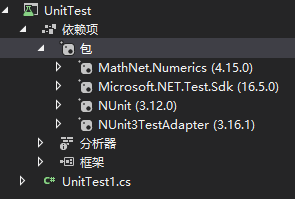using System; using System.Collections.Generic; using System.Linq; using MathNet.Numerics.Distributions; using MathNet.Numerics.LinearAlgebra; using NUnit.Framework;
namespace UnitTest {     public class Tests     {
public Matrix<double> Matrix;
[SetUp]         public void Setup()         {             var rnd = new Random();             //随机生成75个浮点数，均值是50，方差是1，这75个数据成正态分布。             var list = Normal.Samples(rnd, 50, 1).Take(75).ToList();             //保留3位小数，保存为数组             var array = list.Select(p => Math.Floor(p * 1000) / 1000).ToArray();             //将数组生成25组，每组3个数的矩阵             Matrix = CreateMatrix.Dense(25, 3, array);             //保存为二维数组的形式 double[][]             var matrix = Matrix.ToRowArrays();         }
[Test]         public void Test1()         {             Assert.AreNotEqual(null, Matrix);             foreach (var rows in Matrix.EnumerateRows())             {                 Console.WriteLine(string.Join(" , ", rows));             }         }
[Test]         public void Test2()         {             Assert.AreNotEqual(null, Matrix);             //子矩阵             var subMat = Matrix.SubMatrix(15, Matrix.RowCount - 15, 0, 3);             foreach (var rows in subMat.EnumerateRows())             {                 Console.WriteLine(string.Join(" , ", rows));             }
Console.WriteLine("-----------------");             //将subMat扩展到Matrix的下方             var stackMat = Matrix.Stack(subMat);             foreach (var rows in stackMat.EnumerateRows())             {                 Console.WriteLine(string.Join(" , ", rows));             }         }
[Test]         public void Test3()         {             //对于一阶线性方程组
//5 * x + 2 * y - 4 * z = -7
//3 * x - 7 * y + 6 * z = 38
//4 * x + 1 * y + 5 * z = 43
//创建方程向量的集合             var vector1 = CreateVector.Dense(new double[] { 5.00, 2.00, -4.00 });             var vector2 = CreateVector.Dense(new double[] { 3.00, -7.00, 6.00 });             var vector3 = CreateVector.Dense(new double[] { 4.00, 1.00, 5.00 });             var vectors = new List<Vector<double>> { vector1, vector2, vector3 };             //方程等号左侧的已知数矩阵             var matrixA = CreateMatrix.DenseOfRowVectors(vectors);             foreach (var rows in matrixA.EnumerateRows())             {                 Console.WriteLine(string.Join(" , ", rows));             }             //方程等号右侧的已知数向量             var vectorB = CreateVector.Dense(new[] { -7.0, 38.0, 43.0 });             //求解             var resultX =matrixA.Solve(vectorB);
Console.WriteLine("结果：");             resultX.ToList().ForEach(p => Console.WriteLine(Math.Round(p, 2)));
}     } }


展开全文矩阵
• 本人在学习过程中发现，随着MathNet的版本更新，很多代码已经失效了，或者是换了个名字，因此写下笔记供大家分享 注意下面大部分代码已经经过验证，可以运行 可能会更新下去？ 一、安装方法 直接输入安装 PS:该安装...
前言
转载至：https://blog.csdn.net/c914620529/article/details/50393223 本人在学习过程中发现，随着MathNet的版本更新，很多代码已经失效了，或者是换了个名字，因此写下笔记供大家分享 注意下面大部分代码已经经过验证，可以运行
可能会更新下去？
一、安装方法
直接输入安装 PS:该安装方法只适用于当前项目，也就是说创建了新的项目，还需再次安装
Install-Package MathNet.Numerics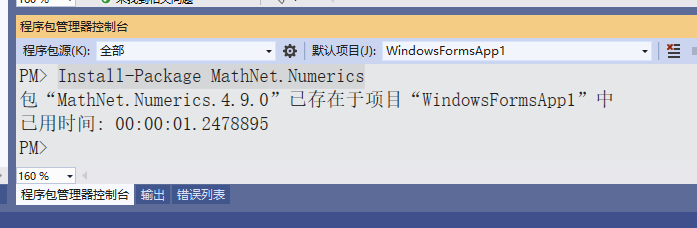二、学习笔记
1.矩阵的定义和初始化
		//3维方阵
var matrix2 = new DenseMatrix(3);
//2*3矩阵
var matrix3 = new DenseMatrix(2, 3);
//5维单位矩阵
var matrixI = DenseMatrix.CreateIdentity(5);

richTextBox1.AppendText(matrixI.ToMatrixString());//直接输出矩阵
richTextBox1.AppendText(matrixI.ToString());//在矩阵输出之前会有一段描述

//可以利用C#中的double[,]创建=====》对应着下图1
double[,] d_matrix =
{
{11,12,13,14,15,16 },
{21,22,23,24,25,26 },
{31,32,33,34,35,36 },
{41,42,43,44,45,46 },
{51,52,53,54,55,56 },
{61,62,63,64,65,66 }
};
var matrix1 = DenseMatrix.OfArray(d_matrix);
richTextBox1.AppendText(matrix1.ToString());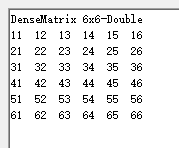2.矩阵操作
        //取第2行开始的2行，第3列开始的3列子矩阵
var m0 = matrix.SubMatrix(2, 2, 3, 3);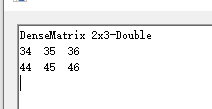//取从第2行第3列开始的3个行元素
var row = matrix.Row(2, 3, 3);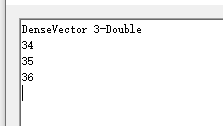//取从第2列第3行开始的3个列元素
var col = matrix.Column(2, 3, 3);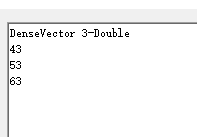//取矩阵的对角线元素向量
var m4 = matrix.Diagonal();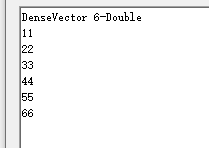double[] d_vector = { 0, 0, 0, 0, 0, 0};
var vector = new DenseVector(d_vector);

//将向量vector插入到指定的行 / 列，原有的行列顺延
var result = matrix.InsertColumn(3, vector);
var result = matrix.InsertRow(3, vector);

PS:插入的向量长度必须和矩阵的行数或列数一样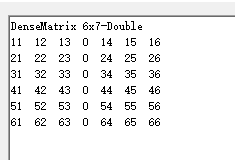//用vector替换指定的行 / 列
matrix.SetColumn(2, (Vector)vector);
matrix.SetRow(3, (double[])vector);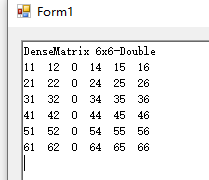//用矩阵替换指定位置的块矩阵
matrix.SetSubMatrix(1, 3, 1, 3, DenseMatrix.CreateIdentity(3));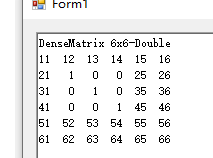//替换矩阵的对角线元素
matrix.SetDiagonal(new[] { 0.0, 0.0, 0.0, 0.0, 0.0, 0.0 });

//将matrixB扩展到matrix的右方 / 上方，将结果保存在result中
matrix.Append(matrixB, result);
matrix.Stack(matrixB, result);

PS:矩阵的大小必须严格设置，matrixB的行数或列数必须和matrix的也一样
        //互换矩阵的3，4行
var permutations = new MathNet.Numerics.Permutation(new[] { 0, 1, 3, 2, 4,5 });
matrix.PermuteRows(permutations);

//互换矩阵的1、2列，3、5列
var permutations = new MathNet.Numerics.Permutation(new[] { 1, 0, 4, 3, 2, 5 });
matrix.PermuteColumns(permutations);

3.矩阵的运算
        //点乘
var result = mA.PointwiseMultiply(mB);

//求逆
var mB = mA.Inverse();

//求解线性方程组，其中m1对应系数矩阵
var m = m1.LU().Solve(m2);

//PS:以下代码只作记录，还未验证！
//行列式
var m = m1.Determinant()
//秩
var m = m1.Rank();
//条件数
var m = m1.ConditionNumber();
//Trace
var m = m1.Trace();
//范数
var m = m1.L1Norm()/L2Norm()/FrobeniusNorm()/InfinityNorm();

展开全文C#
• 最新的C# 数学开源库，VS2010打开直接编译，工程我已经修改，不用作任何改动就能用
• MathNet是在.net编程中很好的一个数据处理的库，其中包含诸如傅里叶变换之后的很多的工具 但是注意 MathNet.Numerics.IntegralTransforms.Fourier.Forward(X,MathNet.Numerics.IntegralTransforms.FourierOptions....
MathNet是在.net编程中很好的一个数据处理的库，其中包含诸如傅里叶变换之后的很多的工具
但是注意
MathNet.Numerics.IntegralTransforms.Fourier.Forward(X,MathNet.Numerics.IntegralTransforms.FourierOptions.Matlab);
这个语句和matlab中的fft(X)是等价的
但是需要注意的是C#中的X需要新建成Complex32类型，并且MathNet.Numerics.IntegralTransforms.FourierOptions.Matlab不能少
展开全文• ## mathNet基础用法

千次阅读 2016-11-04 11:36:47
// // Math.NET Numerics, part of the Math.NET Project // http://numerics.mathdotnet.com // http://github.com/mathnet/mathnet-numerics // http://mathnetnumerc#
• https://www.nuget.org/packages/MathNet.Numerics/ 下载.nupkg文件键，visual studio中打开Nuget，选择程序包源为下载的nupkg所在的文件夹 安装Math.Net.Numeric包 using System; using System.Collections....c#
• C#开发的数学计算方法，包括线性代数、方程组求解
• Math.net计算库是专为.NET开发的开源科学计算库。包含了大部分矩阵运算、线性运算等功能。 1.安装 项目 - 右键 - 管理NuGet程序包 2.矩阵定义 //矩阵的定义和初始化 var matrix1 = new DenseMatrix(3);...va...矩阵加减乘除 矩阵定义
• 因为要计算矩阵与向量的乘法 所以我在vs里使用了MathNet 直接在NuGet里面下载就可以 下载完只后会遇到一个问题 因为Unity3d项目里面没有MathNet，所以会报错，这时需要我们把与MathNet有关的.dll文件复制后放入Unity...unity3d
• //将mathnet的两个引用注释掉，不用函数就可以运行 ` using System; using UnityEngine; using MathNet.Numerics.Integration; using MathNet.Numerics; public class trail : MonoBehaviour { ...c# c#...